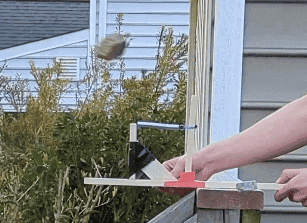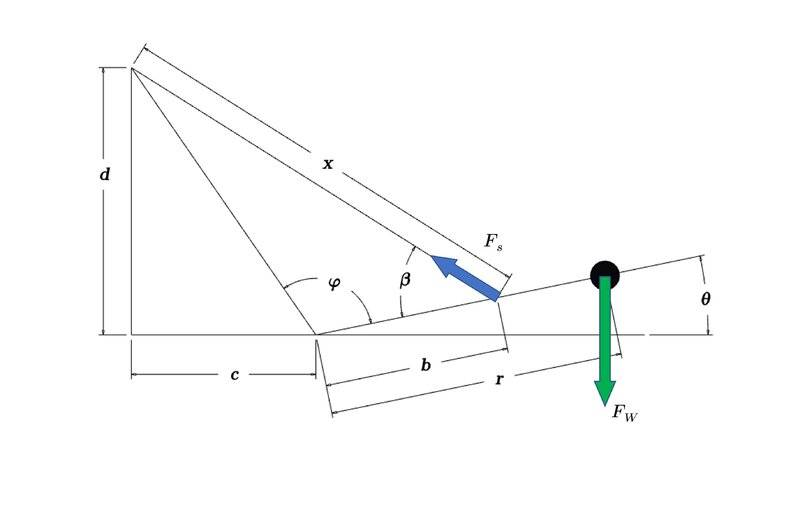# Calculating the Distance that a Catapult can launch a Projectile

• B
GopherTv
TL;DR Summary
How do I calculate the distance to which a catapult can launch rocks of different mass?I know the spring constant of my spring, 90.54 n/m
The spring is stretched 13.5 cm
Im launching of a deck that is 1.75 meters high

Homework Helper
Gold Member
How @GopherTv and welcome to PF.

How about measuring the distance? What is calculating going to do for you that measuring won't. If you want to calculate a number using a formula, at the very least you will need to know the speed and the angle at which the rock leaves the catapult. Also, it looks like you are shooting this out a window, so you will need to know the vertical height from the initial position of the rock to the ground.

•erobz
I know the spring constant of my spring, 90.54 n/m
The spring is stretched 13.5 cm
Calculate the energy stored in the spring that will become kinetic energy.
Weigh the rock, compute the initial velocity for that rock.
Measure the launch angle. Predict the trajectory.

•hutchphd
GopherTv
Calculate the energy stored in the spring that will become kinetic energy.
Weigh the rock, compute the initial velocity for that rock.
Measure the launch angle. Predict the trajectory.
Should I account for the rotational energy of the lever arm or will that be very little

Should I account for the rotational energy of the lever arm or will that be very little
The accuracy of the prediction will be dependent on how much energy accounting you do.
If you avoid computation by experimental calibration, then you could avoid much of the physics. You might then need to use standard rocks.

Homework Helper
Also, it looks like you are shooting this out a window
OP says that it is off a deck. The diagonal members on the bottom which you are taking to be the sill of a window appear to be the railing of a stairway leading down from the deck. He is launching from the deck railing. The corner of the window top that you imagine appears to be the curve of the downspout for the roof gutter.

Gold MemberSumming the torques about the point I forgot to label ( also I didn't include the force of the weight of the rod in the diagram ):

$$b F_s \sin \beta - r m g \cos \theta - \frac{r}{2} m_r g \cos \theta = \left( I_{r_G} + m_r \left( \frac{r}{2} \right)^2 + m r^2 \right) \frac{ d \omega }{dt}$$

Where;

## I_{r_G} ## is the mass moment of inertia (M.o.I) of the rod about its CM
## m_r ## is the mass of the rod

$$F_s = k ( x - x_o )$$

$$\sin \beta = \frac{ \sqrt{d^2 + c^2} \sin \varphi }{x}$$

$$\varphi = \pi - \left( \theta + \arctan \left( \frac{d}{c} \right) \right)$$

and

$$x = \sqrt{ d^2 + c^2 + b^2 - 2 b \sqrt{d^2 + c^2} \cos \varphi }$$

You'll want to integrate this numerically to determine the angular velocity until the angel of launch (it should be close to ## \pi/2 ## ), and then extract the tangential velocity from:

$$v = r \omega$$

From there its kinematics (ignoring air resistance )

Like I said, not an analytical result, but you can program it without much difficulty. If you have questions please feel free to comment.

Last edited: